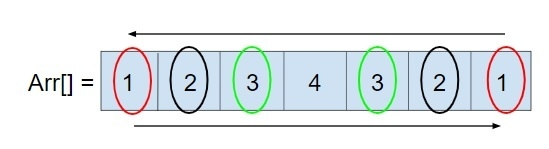# C Program to check if an array is palindrome or not using Recursion

Given an array arr[n] where n is some size of an array, the task is to find out that the array is palindrome or not using recursion. Palindrome is a sequence which can be read backwards and forward as same, like: MADAM, NAMAN, etc.

So to check an array is palindrome or not so we can traverse an array from back and forward like −In recursion also we have to change the start and end value till they are equal or when the values as start and end are not equal then exit and return false that the given array is not palindrome.

## Example

Input: arr[] = { 2, 3, 4, 3, 2}
Output: Yes, the array is Palindrome
Explanation: the array is identical from start (2, 3, 4, 3, 2) and end (2, 3, 4, 3, 2).
Input: arr[] = {1, 2, 3, 4}
Output: No, the array is not Palindrome
Explanation: The array is not identical from start (1, 2, 3, 4) and end (4, 3, 2, 1).

Approach used below is as follows

We will recursively do the following steps −

• Check arr[start] is equal to arr[end] and start < end
• Goto step 1.

## Algorithm

Start
In function int palindrome(int arr[], int start, int end)
Step 1-> If start >= end then,
Return 1
Step 2-> If arr[start] == arr[end] then,
Return palindrome(arr, start + 1, end - 1)
Step 3-> Else {
Return 0
In fucntion int main()
Step 1-> Declare and initialize an array arr[]
Step 2-> Declare and initialize n = sizeof(arr) / sizeof(arr
Step 3-> If palindrome(arr, 0, n - 1) == 1 then,
Print "Yes, the array is Palindrome”
Step 4-> Else
Print "No, the array is not Palindrome”
Stop

## Example

#include <stdio.h>
// Recursive pallindrome function that returns 1 if
// palindrome, 0 if it is not
int palindrome(int arr[], int start, int end) {
// base case
if (start >= end) {
return 1;
}
if (arr[start] == arr[end]) {
return palindrome(arr, start + 1, end - 1);
} else {
return 0;
}
// Driver code
int main() {
int arr[] = { 1, 2, 0, 2, 1 };
int n = sizeof(arr) / sizeof(arr);
if (palindrome(arr, 0, n - 1) == 1)
printf("Yes, the array is Palindrome");
else
printf("No, the array is not Palindrome");
return 0;
}

## Output

If run the above code it will generate the following output −

Yes, the array is Palindrome